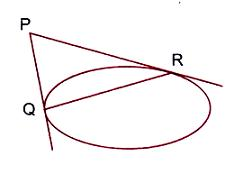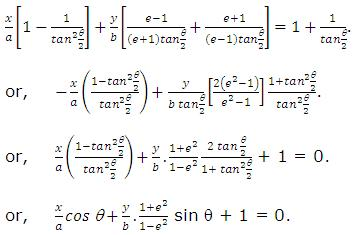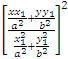×#### Thank you for registering.

One of our academic counsellors will contact you within 1 working day.

Click to Chat

1800-1023-196

+91-120-4616500

CART 0

• 0

MY CART (5)

Use Coupon: CART20 and get 20% off on all online Study Material

ITEM
DETAILS
MRP
DISCOUNT
FINAL PRICE
Total Price: Rs.

There are no items in this cart.
Continue Shopping```Tangent and Normal

Table of Content

How do you find a tangent line to an Ellipse?

Equation of the tangent to ellipse in terms of 'm’

Equation of tangent line to ellipse in different forms

How do you find the equation of the normal to an Ellipse?

Pair of Tangents and Chord of Contact

Related ResourcesThe line y = mx + c meets the ellipse x2/a2 + y2/b2 = 1 in two real, coincident or imaginary points according as c2 < = or > a2m2 + b2.

Hence, y = mx + c is tangent to the ellipse x2/a2 + y2/b2 = 1 if c2 = a2m2 + b2.

The equation of the chord to the ellipse joining two points with eccentric angles α and β is given by

x/a cos ((α + β)/2) + y/b sin ((α + β)/2) = cos ((α - β)/2)

How do you find a tangent line to an Ellipse?

The equation of the chord to the ellipse

The ellipse is x2/a2 +y2/b2 = 1                         ...... (1)

Let P(x1, y1) and Q(x2, y2) be two points on the ellipse. The equation of the line PQ is,

y - y1 = ((y2 - y1)/(x2 - x1)) (x - x1)                 ...... (2)

Since points P and Q lie on (1), we get

(y2 - y1)/(x2 - x1) = (-b2 (x2-x1))/(a2 (y2-y1) )

So (2) becomes

y - y1 = (-b2 (x2-x1))/(a2 (y2 - y1)) (x - x1)

As point Q approaches point P along the ellipse, the line PQ tends to the tangent at P. So, by substituting x1 and y1 for x2 and y2 in the above equation, we have the equation of the tangent at P as

y - y1 = (-b2 (2x1))/(a2 (2y1)) (x - x1)

⇒ (xx1)/a2 + yy1/b2 = (x12)/a2 +(y12)/b2 = 1     [as P lies on (1)]

Hence the equation of the tangent to x2/a2 + y2/b2 = 1 at P(x1,y1) is xx1/a2 + yy1/b2 = 1.            ...... (3)

Equation of the tangent to ellipse in terms of 'm’

Let the line y = mx + c                                                ....... (4)

Touch the ellipse x2/a2 +y2/b2 = 1                                ...... (5)

Eliminating y between (4) and (5), we get;

x2(b2 + a2m2) + 2a2mcx + a2(c2 - b2) = 0             ...... (6)

If (4) touches (5) then the roots of (6) must be coincident i.e. D = 0

i.e. (2a2mc)2 = 4(b2 + a2m2) a2(c2 - b2)

Solving this we get c = +√(a2m2+b2)

So the equation of tangent is y = mx + √(a2m2+b2) for all real m    ......(7)

From the equation (6) and (7) we get the point of contact as ((± a2m)/√(a2m2 + b2 ), (± b2)/√(a2m2 + b2))

Equation of tangent line to ellipse in different forms

As illustrated above, the various tangent lines to an ellipse can be summed as:

Point form: As discussed above, the equation of the tangent to the ellipse x2/a2 + y2/b2 = 1 at the point (x1, y1) is xx1/a2 + yy1/b2 = 1.

Parametric form: The equtaion of the tangent to the ellipse x2/a2 + y2/b2 = 1 at the point (a cos θ, b sin θ) is x cos θ/a + y sin θ/b = 1.

Slope Form: The equation of tangent to ellipse x2/a2 + y2/b2 = 1 in terms of slope is given by y = mx ± √(a2m2 + b2).

In general, the line x cos c + y sin c = l is a tangent if l2 = c2 cos2α + b2 sin2α.

lx + my + n = 0  is a tangent if n2 = a2l2 + b2m2.

How do you find the equation of the normal to an Ellipse?

The normal to a curve is a line perpendicular to the tangent to curve through the point of contact.

As discussed above in case of tanegnts, the various ways of finding the normal are listed below:

A line y = mx + c is normal to the ellipse x2/a2 + y2/b2 = 1 if c2 =  m2 (a2 - b2)2/ (a2 + b2m2)

Point form: The equation of the normal to the ellipse x2/a2 + y2/b2 = 1 at the point (x1, y1) is a2x/x1 - b2y/y1 = a2 - b2.

Parametric form: The equtaion of the normal to the ellipse x2/a2 + y2/b2 = 1 at the point (a cos θ, b sin θ) is ax sec θ - by cosec θ = a2 - b2.

ope Form: The equation of normal to ellipse x2/a2 + y2/b2 = 1 in terms of slope is given by y = mx ± m(a2-b2)/√(a2 + b2m2). The coordinates of the point of contact are (((± a2)/√(a2 + b2m2), (± b2m)/√(a2 + b2m2))

How many tangents can be drawn to an ellipse?

We know that y = mx + √(a2 m2+b2) is a tangent to the ellipse x2/a2 +y2/b2 = 1 for real values of m. If this tangent passes through a point (x1, y1) we have y1 = mx1 + √((a2 m2+b2)).

or m2(x12 - a2) - 2x1y1m + (y12 - b2) = 0, which being a quadratic equation in m gives two values of m. Thus from a point two tangents (corresponding to two values of m) can be drawn to an ellipse.

Pair of Tangents and Chord of Contact

From a fixed point (x1, y1) in general two tangents can be drawn to an ellipse. The equation of the pair of tangents drawn to the ellipse x2/a2 +y2/b2 = 1 is given by (x2/a2 + y2/b2-1)((x12)/a2 +(y12)/b2 - 1) = (xx1/a2 + yy1/b2-1)2.In symbols we write SS1 = T2, where

S ≡ x2/a2 +y2/b2 = 1,  S1≡ (x12)/a2 +(y12)/b2 – 1 and  T ≡ xx1/a2 + yy1/b2 – 1

Key points on chord of contact:

If from the point P(x1, y1) tangents PQ and PR be drawn to the ellipse x2/a2+y2/b2 = 1, then the line joining the points of contact Q and R is called the chord of contact. Equation of the chord of contact is xx1/a2 + yy1/b2 - 1 = 0 or T = 0.

Equation of the Chord Joining the Points (α cosα, β sinα), (α cosβ, β sinβ) is x/a cos((α+β)/2)+ y/b sin((α+β)/2) = cos((α-β)/2)

Equation of a chord which is bisected at the point (x1, y1) is xx1/a2 + yy1/b2 -1 = (x12)/a2 +(y12)/b2 - 1 or T = S1.

To find the length of the chord intercepted by the ellipse x2/a2 + y2/b2 = 1 on the straight line y = mx + c.

Points of intersection of the ellipse and the line are given by x2/a2 +(mx+c)2/b2 = 1

i.e. (a2 m2 + b2)x2 + 2a2cmx + a2(c2 - b2) = 0                ...... (1)

Therefore the straight line meets the ellipse in two points (real, coincident or imaginary).

If (x1, y1) and (x2, y2) be the points of intersection, the length of the chord is

√((x1-x2)2 + (y1-y2)2) = √(1 + m2) = |x1 - x2|             ...... (2)

(since y1 - y2 = m (x1 - x2))

where x1 and x2 are the roots of the equation (1), and

x1 + x2 = -(2a2cm)/(a2 m2 + b2), x1 x2 (a2 (c2 - b2))/(a2 m2 + b2) so that

(x1 - x2)2 = (x1 + x2)2 - 4x1 x2 = (4a2 c2 m2)/(a2 m2 + b2)2 -(4a2(c2 - b2))/((a2m2 + b2)) = (4a2 b2 (a2 m2 + b2 - c2))/(a2 m2 + b2)2.

Hence the length of the chord is √(((1+m2)4a2 b2 (a2 m2 + b2 - c2))/(a2 m2 + b2)2).

i.e. 2ab/(a2 m2 + b2)2) √((1+m2))(a2) m2)+b2)-c2))).

=> e cos(α+β)/2 = cos(α-β)/2

=> e [cos α/2 cos β/2 + sin α/2 sin β/2] = cos α/2 cos β/2 + sin α/2 sin β/2

=> e [1 - tan α/2 tan β/2] = 1 + tan α/2 tan β/2 => tan α/2 . tanβ/2 = (e-1)/(e+1).

If the chord passes through (-ae, 0) then tanα/2 . tanβ/2 =  (e+1)/(e-1).

Illustration:

Find the locus of the point of intersection of the tangents to the ellipse x2/a2+y2/b2 = 1 (a > b) which meet at right angles.

Solution:

The line y = mx ±√(a2 m2+b2) is a tangent to the given ellipse for all m. Suppose it passes through (h, k).

⇒ k - mh = √(a2m2 + b2 ) ⇒ k2 + m2h2 - 2hkm = a2m2 + b2

⇒ m2 (h2 - a2) - 2hkm + k2 - b2 = 0.

If the tangents are at right angles, then m1m2 = -1.

⇒ (k2-b2)/(h2-a2 ) = - 1 ⇒ h2 + k2 = a2 + b2.

Hence the locus of the point (h, k) is x2 + y2 = a2 + b2 which is a circle. This circle is called the Director Circle of the ellipse.

Note:

The locus of the point of the intersection of two perpendicular tangents to an ellipse is a circle known as the director circle.

Illustration:

Prove that the locus of the mid-points of the intercepts of the tangents to the ellipse x2/a2 + y2/b2 = 1 = 1, intercepted between the axes, is a2/x2 +b2/y2 = 4.

Solution:

The tangent to the ellipse at any point (a cosθ, b sinθ)(x cosθ)/a + (y sinθ)/b = 1.

Let it meet the axes in P and Q, so that P is (a secθ, 0)

and Q is (0, b cosecθ). If (h, k) is the mid-point of PQ, then h = (a sec θ)/2.

⇒ cosθ = a/2h and k = (b cosecθ)/2 ⇒ sinθ = b/2k.

Squaring and adding, we get a2/4h2 + b2/(4k2 ) = 1

Hence the locus of (h, k) is a2/x2 + b2/y2 = 4.

Illustration:

Prove that the product of the lengths of the perpendiculars drawn from the foci to any tangent to the ellipse x2/16 + y2/9 = 1 is equal to 9.

Solution:

For the given ellipse a = 4, b = 3 and hence 9 = 16 (1 - e2)

⇒ e = √7/4. The foci are thus located at (√7,0) and (-√7,0).

Equation of a tangent to the given ellipse is

y = mx + √(16m2+9)    (as a = 4, b = 3).

Lengths p1 and p2 of the perpendiculars drawn from the foci are

p1 = (√(16m2 + 9) +√7m)/(1 + m2 ) and p2 = (√(16m2 + 9) - √7m)/(1+m2)

⇒ p1p2 = (16m2 + 9 – 7m2)/(1 + m2) = 9(1 + m2)/(1 + m2) = 9.

Note:

The product of lengths of the perpendiculars drawn from the foci to any tangent to the ellipse x2/a2 +y2/b2 = 1 is b2.

Illustration:

If the normals at the end of a latus rectum of the ellipse x2/a2 +y2/b2 = 1 passes through the extremity of a minor axis, prove that e4+e2-1=0.

Solution:

Equation the normal to te given ellipse at

(ae,b2/a) is (x-ae)/(ae/a2) = (y-b2/a)/(b2/ab2).

Since it passes through (0, - b).

- a2 = - ab - b3

⇒ a2 = ab + a2 (1 - e2)

or b = ae2 ⇒ b2 = a2e4

or a2 (1 - e2) = a2e2 ans so we get a4 + e2 - 1 = 0.

Illustration:

The tangent and normal at a point P on an ellipse meet the minor axis at A and B. Prove that AB subtends a right angle at each of foci.

Solution:

The equations of the tangents and normal at a point P(x1, y1) on the ellipse x2/a2+ y2/b2 = 1 are

xx1/a2 + yy1/b2 = 1                                    ...... (1)

and (x-x1)/((x1/a2)) + (y-y2)/((y1/b2)) = 1       ...... (2)

Also the minor axis is y-axis i.e. x = 0

Solving (1) and x = 0, we have A (0, b2/y1)

Solving (2) and x = 0, we have B (0, y1 - a2y1/b2)

Let S(ae, 0) be one of the foci of the ellipse. Then the slope of SA = ((b2/y1) - 0)/(0-ae)= b2/aey1 = m1 (say)

And the slope of SB = ([y1 (a2/b2 ) y1 ]-0)/(0-ae)

= y1/ae ((a2-b2 ))/b2= (y1 a2 e2)/(aeb2) [·.· b2 = a2(1 - e2)]

= aey1/b2 = m2  (say)

Evidently m1m2 = -1 ⇒ SA | SB

i.e. AB subtends a right angle at S(ae, 0). Similarly we can show that AB subtends a right angle at the other focus S'(-ae, 0).

Illustration:

Prove that the locus of the middle points of the portion of tangent to the ellipse x2/a2 +y2/b2 = (a+b) between the axis is the curve a/x2 + b/y2 = 4/((a+b)).

Solution:

Any tangent to the ellipse  x2/a2 +y2/b2 = 1 , is

y = mx + √(a2 m2 + b2)

Therefore the tangent to given ellipse is

y = x + √(a(a+b) m2+b(a+b))                  ...... (1)

(1)  Meets x-axis at {-√(a(a+b) m2+ b(a+b) )/m,0}

(2)  Meets y-axis at {0,√(a(a+b) m2+b(a+b))}

Let (x1, y1) be the mid points of the portion of the tangent intercepted between the axes, then

x1 = 1/2 [{-√(a(a+b) m2 + b(a+b))/m} + 0]

y1 = 1/2 [0+{√(a(a+b) m2+b(a+b))} + 0]

⇒ 4x12 = (a(a+b) m2+ b(a+b))/m2

and 4y12 = a(a + )m2 + b(a + b)

Eliminating m, we get

a(a+b)/(4x12) - b(a+b)/(4y12) = am2/(am2+b) + b/(am2+b) = 1

.·. The locus of (x1, y1) is a/x2 + b/y2 = 4/(a+b)  = 1

Illustration:

If PS1Q and PS2R be two focal chord of the ellipse whose two foci are S1 and S2and the eccentric angle of P is 'θ' then show that the equation of chord QR is x/a cos θ + y/b.(1+e2)/(1-e2) sin θ + 1 = 0.

Solution:

Let Q be (a cos α, b sin α) and R be (a cos β, b sin β) then the equation of the chord QR is

x/α cos(α+β)/2 + y/b sin(α+β)/2 = cos(α-β)/2

which on simplifying becomes

x/a (1-tan α/2 tan β/2)+y/b (tan α/2+tan β/2) = 1 + tan α/2 tan β/2 ..... (1)

Also, PQ and PR are focal chord thus

tan θ/2 tan α/2 = (e-1)/(e+1) and tan θ/2 tan β/2 = (e+1)/(e-1)

(From previous illustration)

On substituting the values of tan α/2  and β/2  in (1), we getIllustration:

Show the locus of middle points chord of the ellipse  x2/a2 +y2/b2 = 1 which subtend right angle at the centre is x2/a4 + y2/b4 =(1/a2 +1/b2 ) (x2/a2 + y2/b2)2.

Solution:

Let (x1, y1) be the middle point of chord PQ, then its equation is

T = S1 or 1/a2 + yy1/b2 =(x1/b2)/a2 +(y1/b2)/b2            ...... (1)

Since the origin 'O' is the centre so the equation of pair of lines OP and OQ can be obtained by homogenizing the equation of the ellipse x2/a2 +y2/b2 = 1, with the help of (1), thus

x2/a2 +y2/b2 = (1)2 =or ((x12)/a2 +(y12)/b2)2 (x2/a2 +y2/b2 ) = (x2 x12)/a4 +(y2 y12)/b4 + (2xyx1 y1)/(a2b2).

It represents a pair of perpendicular lines if

1/a2 ((x12)/a2 +(y12)/b2 )2 - (x12)/a4 + 1/b2 ((x12)/a2 +(y12)/b2)2-(y12)/b4 = 0.

or, (x12)/a4 +(y12)/b4 = (1/a2 +1/b2)(x2/a4 +y2/b4)2.

So the locus of (x1, y1) is

x2/a4 + y2/b4 = (1/a2 + 1/b2 ) (x2/a2 +y2/b2 )2.

Related Resources

For getting an idea of the type of questions asked, refer the previous year papers.

Fast Track your revision with the ultimate Revision Notes on Ellipse.

To read more, Buy study materials of Ellipse comprising study notes, revision notes, video lectures, previous year solved questions etc. Also browse for more study materials on Mathematics here.
```### Course Features

• 731 Video Lectures
• Revision Notes
• Previous Year Papers
• Mind Map
• Study Planner
• NCERT Solutions
• Discussion Forum
• Test paper with Video Solution